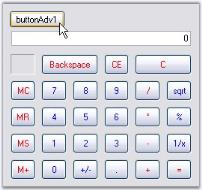# Pop-Up CalculatorControl

8 Jun 20211 minute to read

The PopupCalculator class can be used to display a popup Calculator control. This class can be created programmatically.

The PopupCalculator control lets you embed a Calculator control object to a button for example. Drop the button onto the form and add the following code snippet.

``````private Syncfusion.Windows.Forms.Tools.PopupCalculator popupCalculator1;
private void buttonAdv1_Click(object sender, EventArgs e)
{

// Create the Popup Calculator.
popupCalculator1 = new Syncfusion.Windows.Forms.Tools.PopupCalculator();

// The control that will act as the Popup's parent.
this.popupCalculator1.ParentControl = this.button1;

// Set the alignment.
this.popupCalculator1.PopupCalculatorAlignment = Syncfusion.Windows.Forms.Tools.CalculatorPopupAlignment.Right;

// Display the Calculator control.
this.popupCalculator1.DisplayCalculator(Point.Empty);

//Sets the size of the calculator
this.popupCalculator1.Size = this.calculatorControl1.Size;
}``````
``````Private popupCalculator1 As Syncfusion.Windows.Forms.Tools.PopupCalculator
Private Sub buttonAdv1_Click(ByVal sender As Object, ByVal e As EventArgs)

' Create the Popup Calculator.
popupCalculator1 = New Syncfusion.Windows.Forms.Tools.PopupCalculator()

' The control that will act as the Popup's parent.
Me.popupCalculator1.ParentControl = Me.button1

' Set the alignment.
Me.popupCalculator1.PopupCalculatorAlignment = Syncfusion.Windows.Forms.Tools.CalculatorPopupAlignment.Right

' Display the Calculator control.
Me.popupCalculator1.DisplayCalculator(Point.Empty)

'Sets the size of the calculator
Me.popupCalculator1.Size = Me.calculatorControl1.Size
End Sub``````